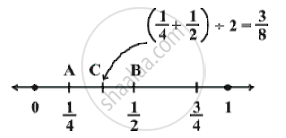Share

# Rational Numbers Between Two Rational Numbers

#### notes

If we write -1/10 as -10000/100000 and 3/10 as 30000/100000 ,

we get the rational numbers -9999/100000 , -9998 / 100000 ,

..., -29998/100000 , 29999/100000 , between -1/10 and 3/10.

For example :  Find a rational number between 1/4 and 1/2.

=>  We find the mean of the given rational numbers.

(1/4 + 1/2)÷ 2 = ((1+2)/4)÷2 = 3/4 xx 1/2 = 3/8

3/8 lies between 1/4 and 1/2.

This can be seen on the number line also.We find the mid point of AB which is C, represented by (1/4 + 1/2) ÷ 2 = 3/8.

### Shaalaa.com

Rational Numbers between two Rational Numbers [00:06:13]
S
0%

S